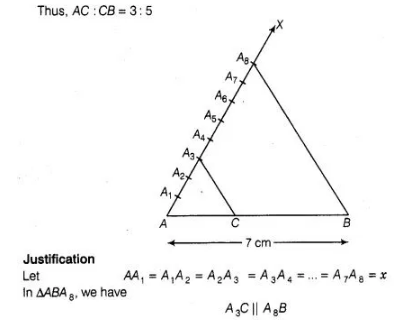# Draw a line segment of length 7 cm.

Question:

Draw a line segment of length 7 cm. Find a point P on it which1 divides it in the ratio 3:5.

Solution:

Steps of construction

1. Draw a line segment AB = 7 cm.
2. Draw a ray AX, making an acute ∠BAX
3. Along AX, mark 3+ 5= 8 points
A1, A2, A3, A4, A5, A6, A7, A8 such that
AA= A1A= A2A3 = A 3A4 = A 4A5 = A 5A6 = A 6= A 7A8
4. Join   A8B
5. From A3, draw A3C || A8B meeting AB at C.
[by making an angle equal to ∠BA 8A at A 3]

Then, C is the point on AB which divides it in the ratio 3 : 5,$\therefore$ $\frac{A C}{C B}=\frac{A A_{3}}{A_{3} A_{8}}=\frac{3 x}{5 x}=\frac{3}{5}$

Hence, $A C: C B=3: 5$